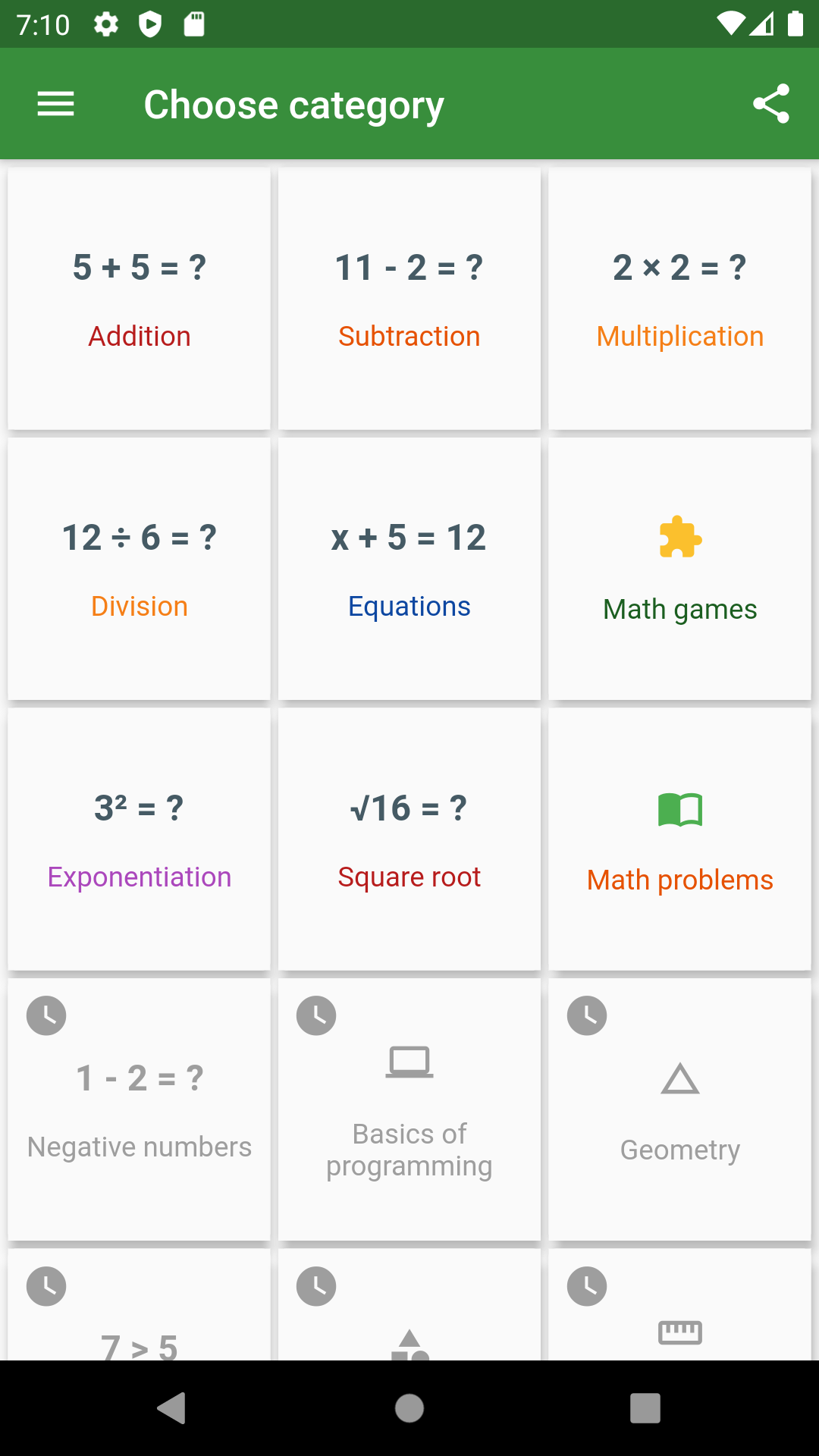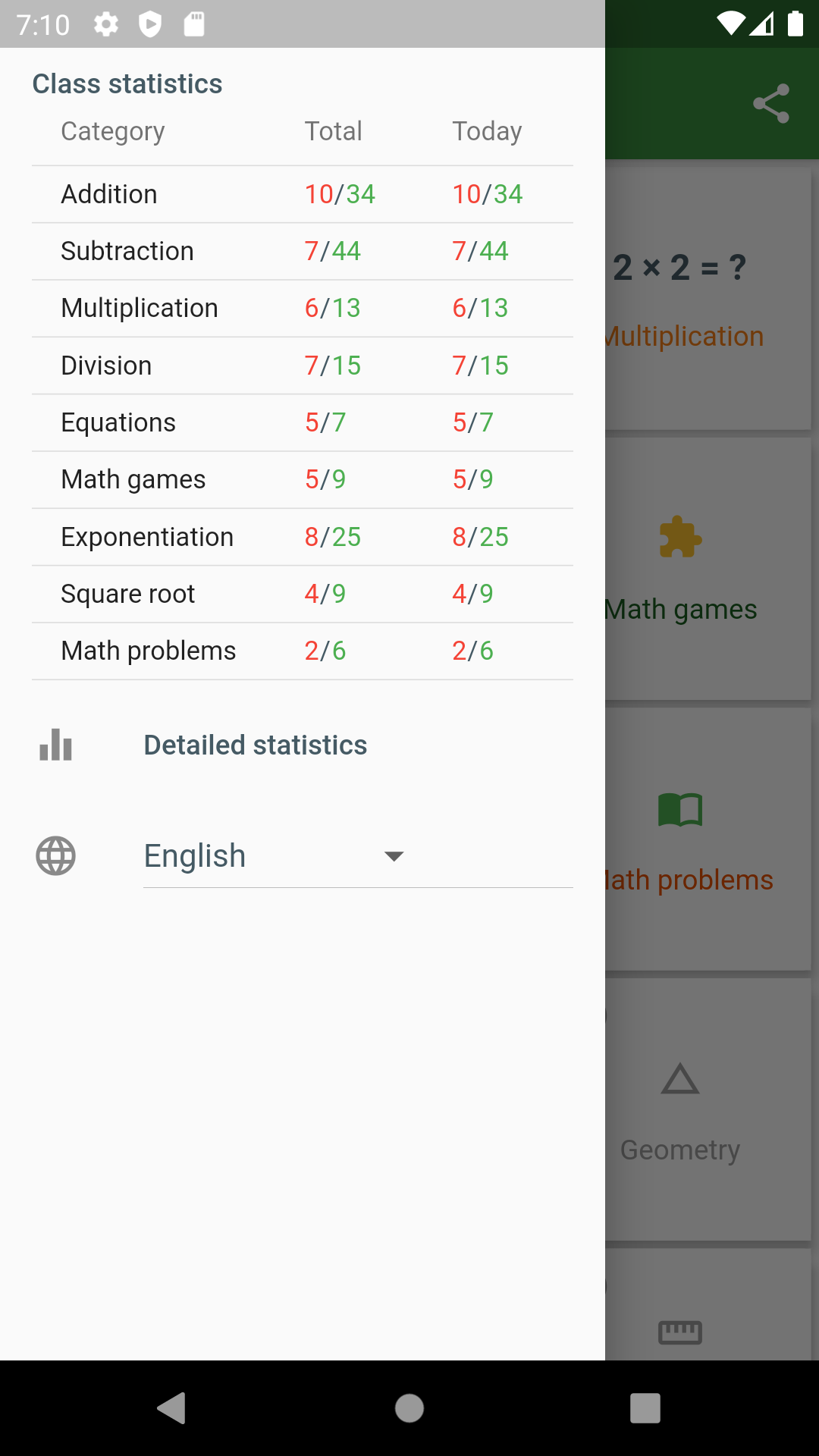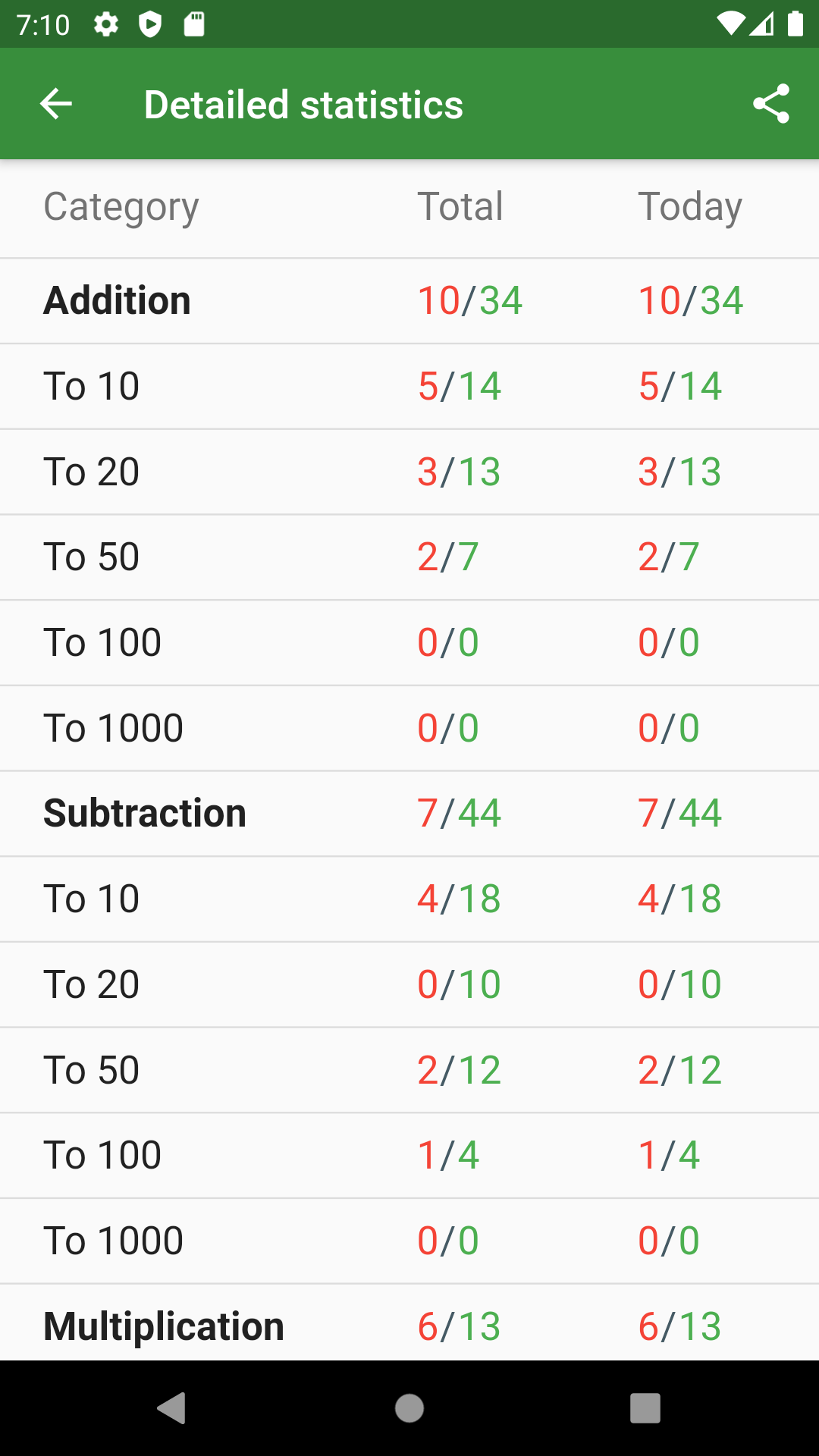MADE WITH    BY THE FLUTTER COMMUNITY
An open list of apps built with Flutter
Feel free to add an app in progress and update it when it goes live## Math Training    Education

App to Improve Math Skills

🎓 Help children and adults develop Math skills.
- Understanding size, shape, and patterns
- Ability to count verbally (first forward, then backward)
- Recognizing numerals
- Identifying more and less of a quantity
- Understanding one-to-one correspondence (i.e., matching sets, or knowing which group has four and which has five)

💾 Memory training.

🧮 Mental math.

🔄 Update and refresh math knowledge.

❎ Multiplication table.

📈 Statistics of correctly and incorrectly solved examples and tasks for the current day and for all time.

💪 Prepare to solve the exam, prepare for the test in mathematics, solve the exam.

Improve math skills in the following categories:

➖ Subtraction
✖️ Multiplication
➗ Division

Equation solving
Exponentiation
Root extraction

📚 Solving math problems

Negative numbers
Percentage
Inequalities

Distance, speed, time (in development)
Fractions (in development)
📐 Geometry (in development)

Area, perimeter and volume (in development)
📏 Unit of measurement (in development)
💡 Logical problem solving (in development)

Algebra (in development)
Trigonometry (in development)
Statistics (in development)
Mathematical analysis (in development)

Certain categories (in development):

Also in the near future it is planned to add such important categories as:

Basics of programming
Preparation for the exam
ACT prep
SAT prep
IQ test

🎮 Math games:

"Find a pair 🧩" is an exciting mathematical game that improves not only the ability to quickly count, but also strengthens the memory of the player.

At the moment, app supports following languages:
🇺🇸 English,
🇮🇱 Hebrew,
🇷🇺 Russian.